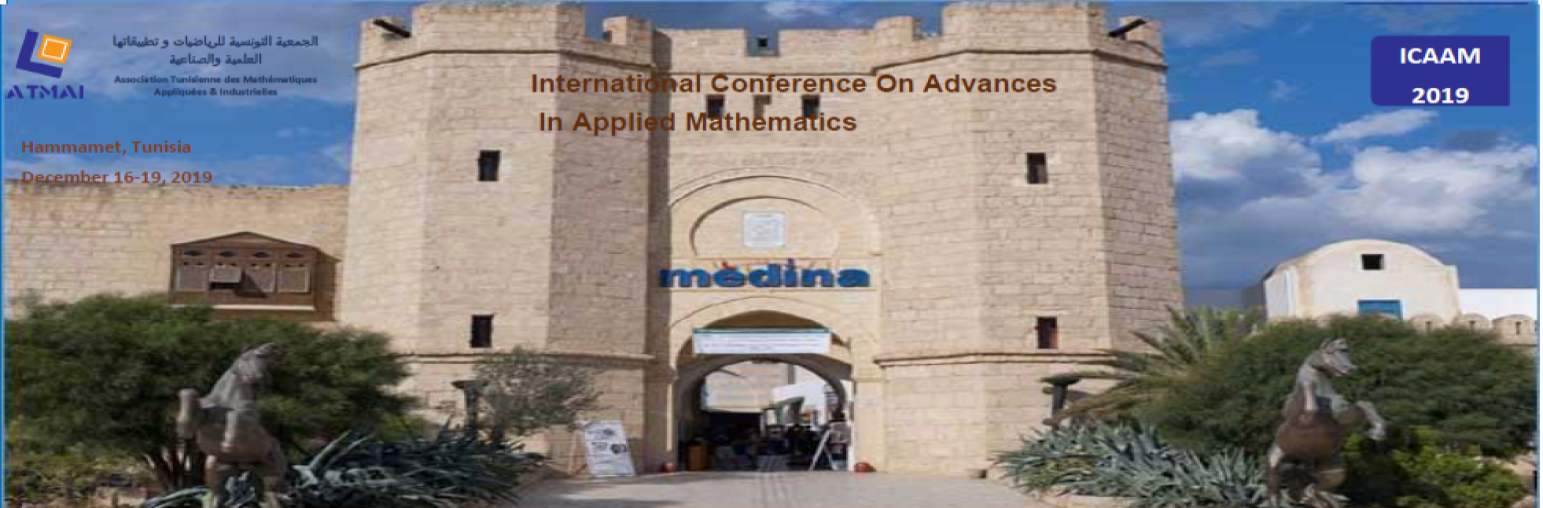### International Conference on Advances in Applied Mathematics ICAAM - 2019

#### Topics

The purpose of this conference is to highlight some of the major theoretical advances and applications in the fields of:
• Control Theory
• Dynamical Systems
• Fixed point theory
• Homogenization
• Inverse Problem
• Nonlinear analysis
• Nonlinear Systems and Matrix
• Numerical Analysis
• Operator Theory
• Ordinary Differential Equations
• Partial Differential Equations
• Probability and Statistics
• Spectral Theory
• Stochastic analysis